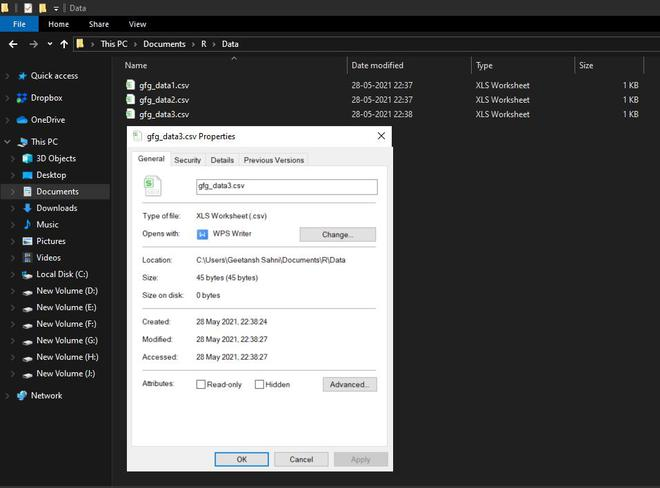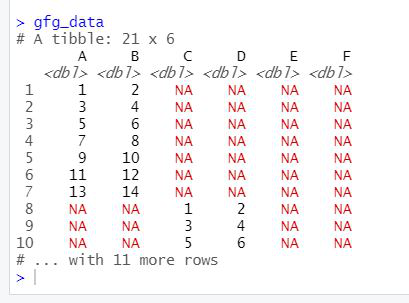# Merge multiple CSV files using R

• Last Updated : 06 Jun, 2021

In this article, we will be looking at the approach to merge multiple CSV files in the R programming language.

In this approach to merge multiple CSV files, the user needs to install and import three different packages namely- dplyr,plyr, and readr in the R programming language console to call the functions which are list.files(), lapply(), and bind_rows() from these packages and pass the required parameters to these functions to merge the given multiple CSV files to a single data frame in the R programming language.

### Function Used

• list.files() function produces a character vector of the names of files or directories in the named directory.

Syntax:

list.files(path = “.”, pattern = NULL, all.files = FALSE,full.names = FALSE, recursive = FALSE, ignore.case = FALSE, include.dirs = FALSE, no.. = FALSE)

• lapply() function returns a list of the same length as X, each element of which is the result of applying FUN to the corresponding element of X.

Syntax:

lapply(X, FUN, …)

• bind_rows() function is an efficient implementation of the common pattern of do.call(rbind, dfs) or do.call(cbind, dfs) for binding many data frames into one.

Syntax:

bind_rows(…, .id = NULL)

Folder in Use:To actually merge multiple CSV/Excel files as one dataframe first the required packages are imported and then list of files are read and joined together.

Example:

## R

 `library``(``"dplyr"``)                                                ``library``(``"plyr"``)                                                 ``library``(``"readr"``)  `` ` `gfg_data <- ``list.files``(path = ``"C:/Users/Geetansh Sahni/Documents/R/Data"``,    ``                       ``pattern = ``"*.csv"``, full.names = ``TRUE``) %>% ``  ``lapply``(read_csv) %>%                                           ``  ``bind_rows                                                      `` ` `gfg_data `

Output:My Personal Notes arrow_drop_up